Constructing a copy of a given angle

Practical Geometry
Concept wise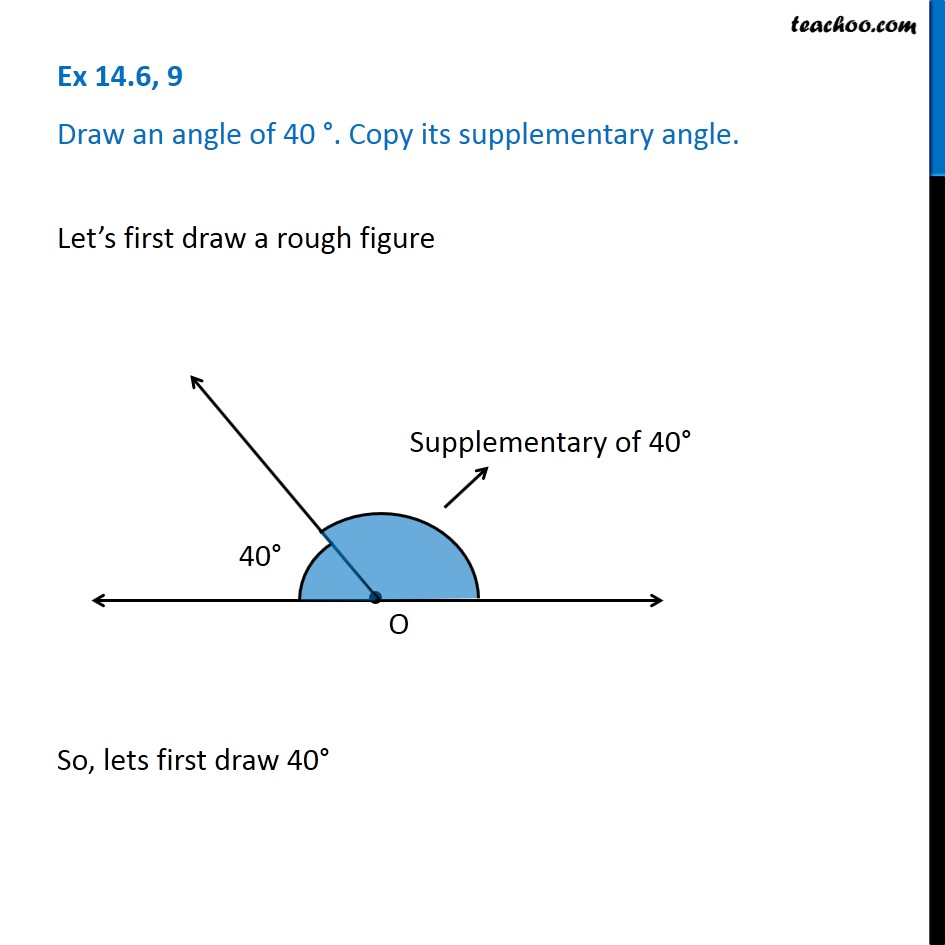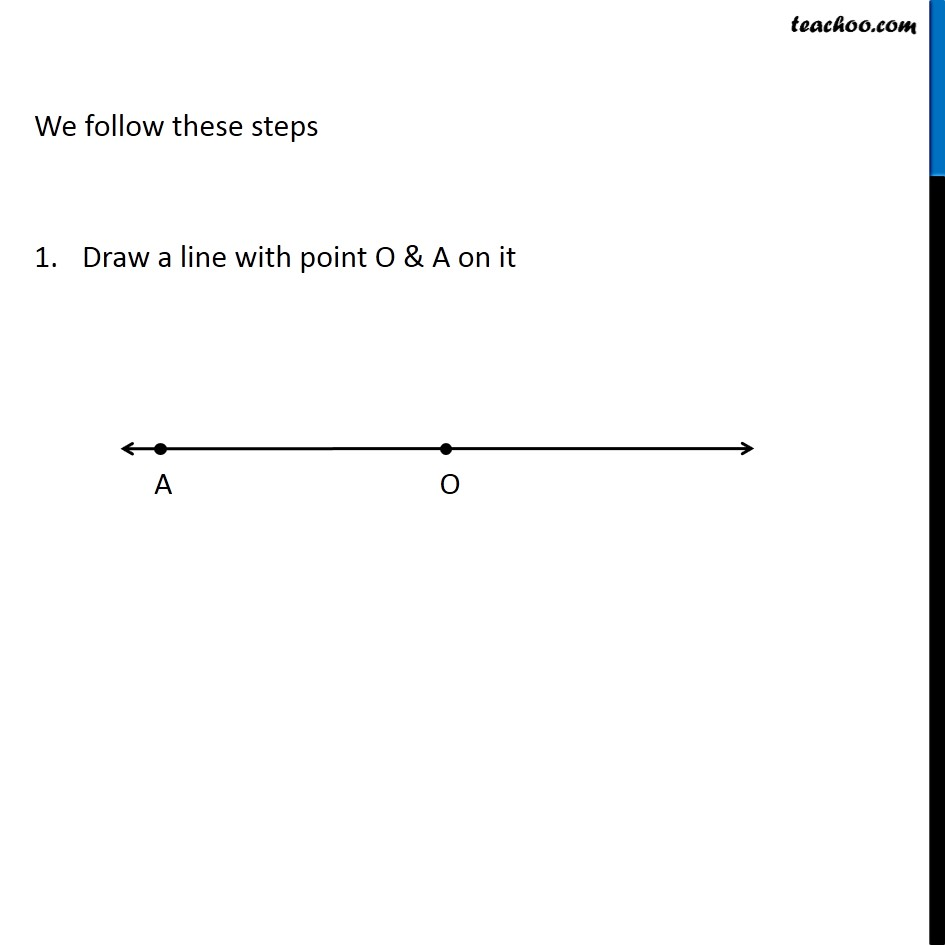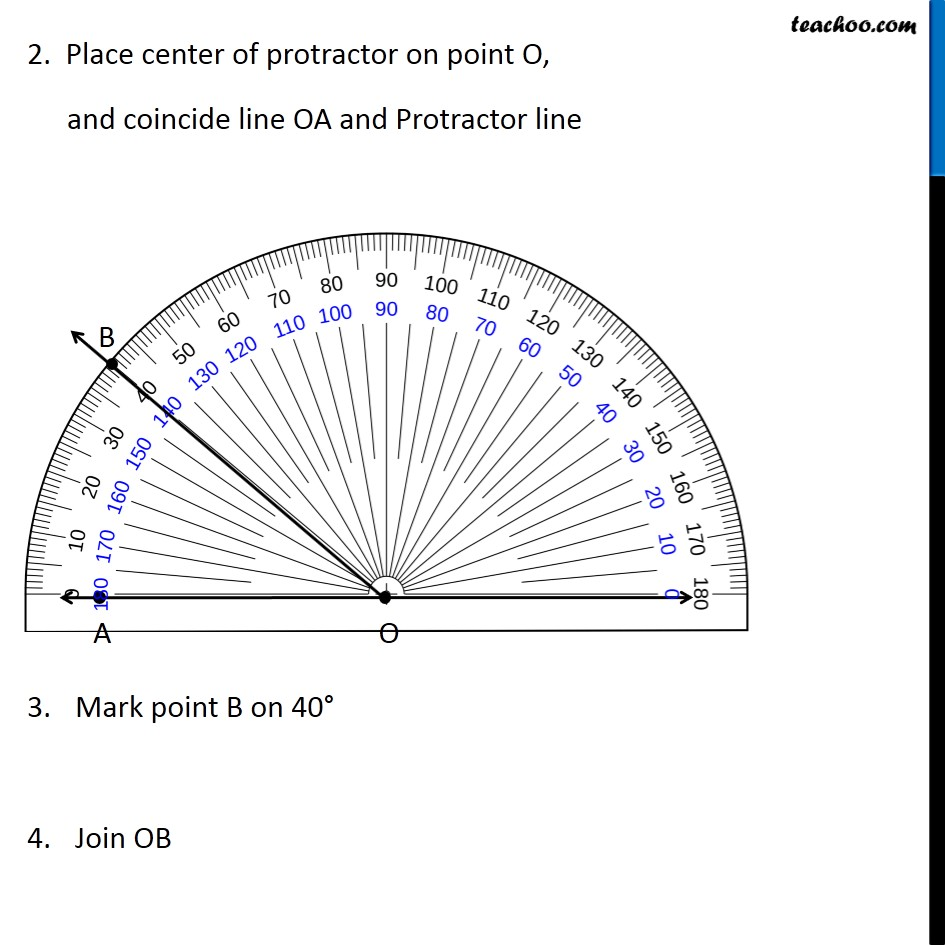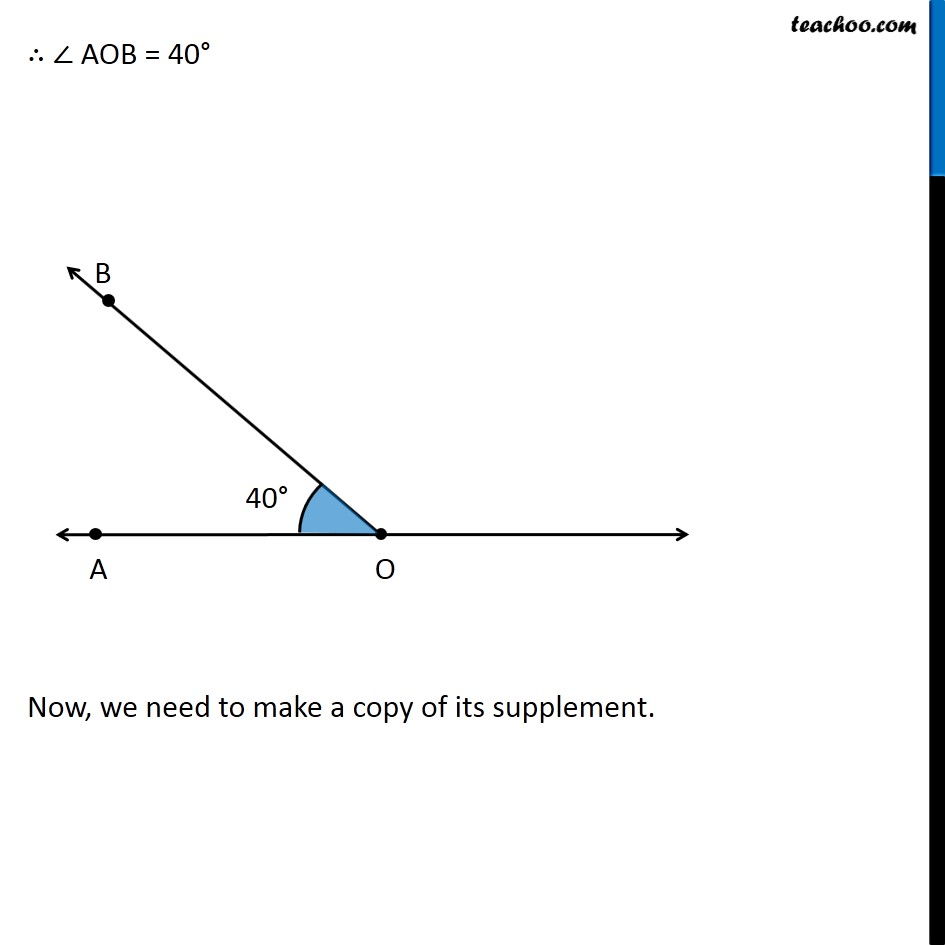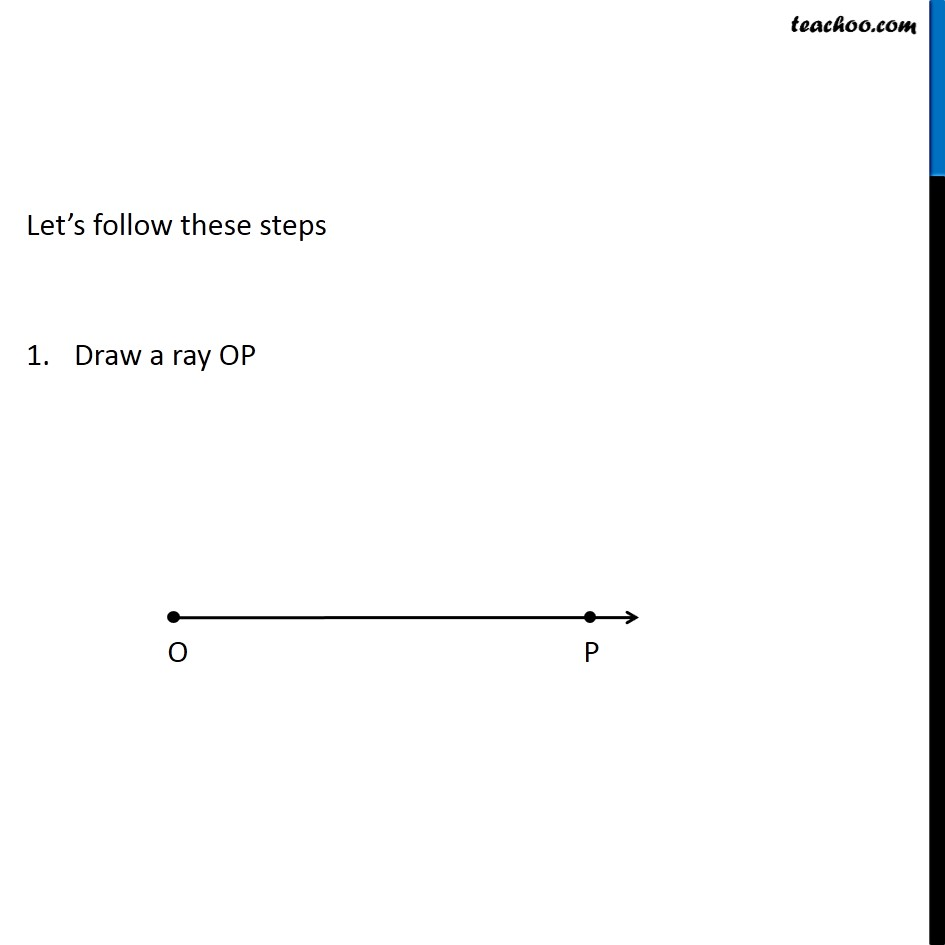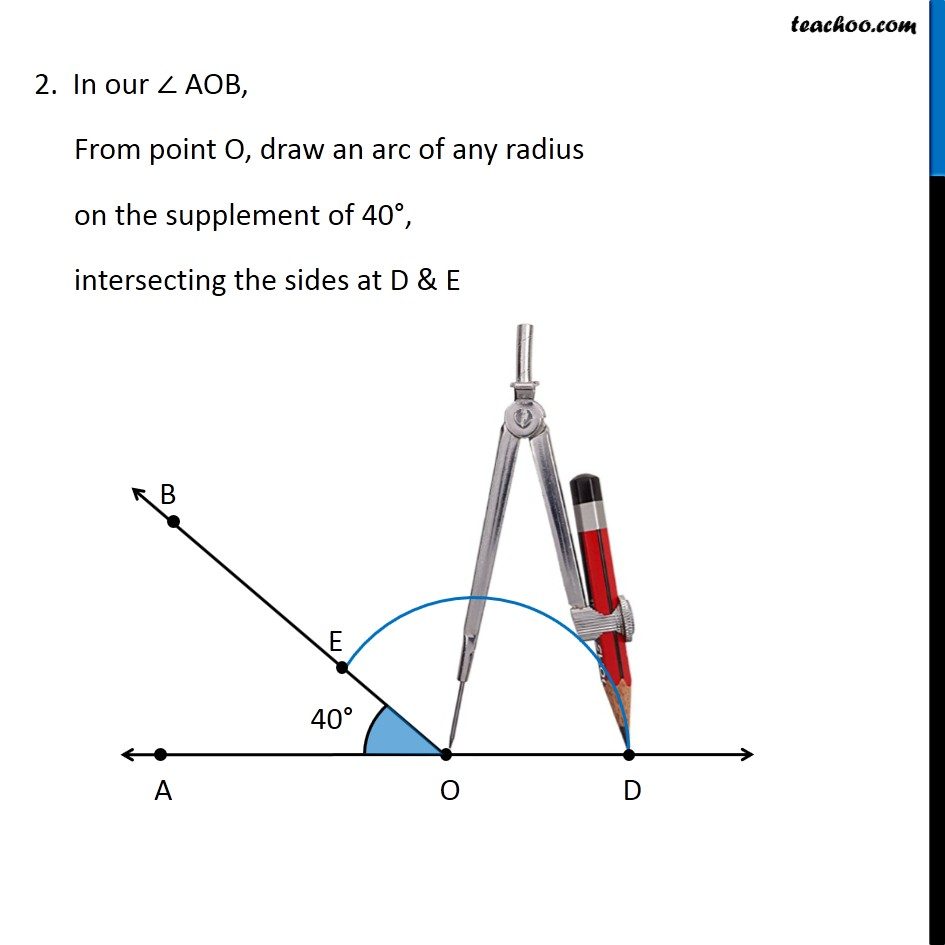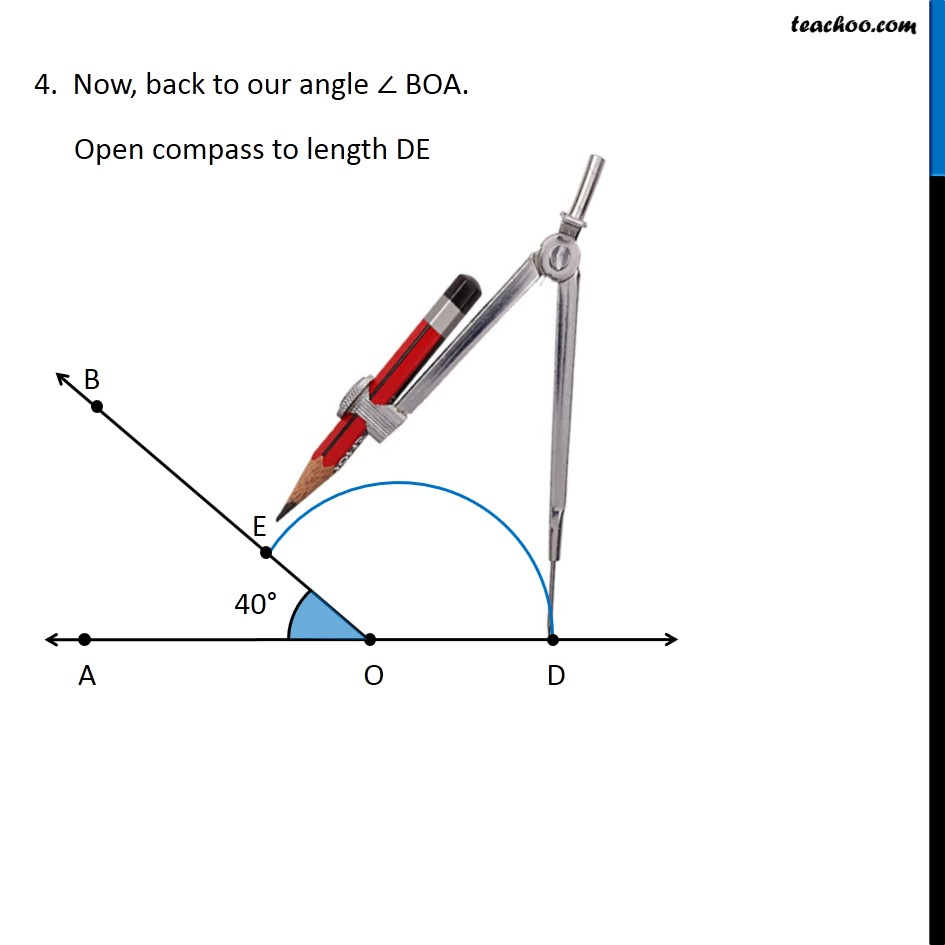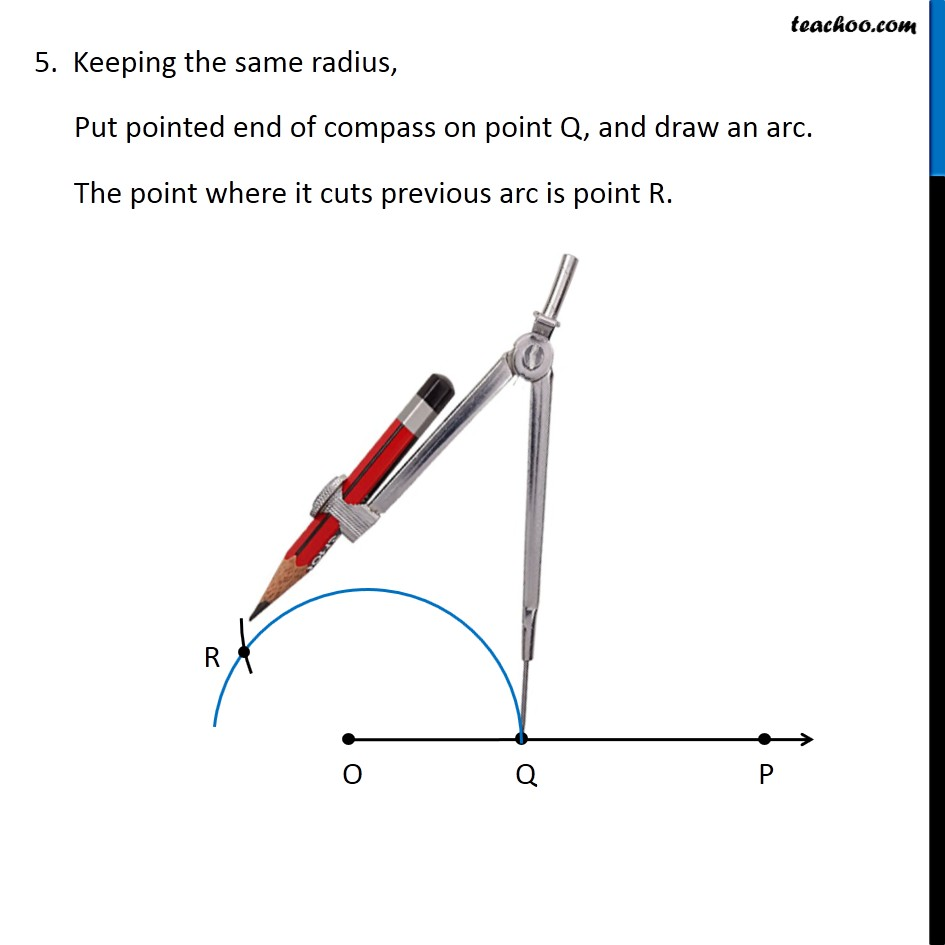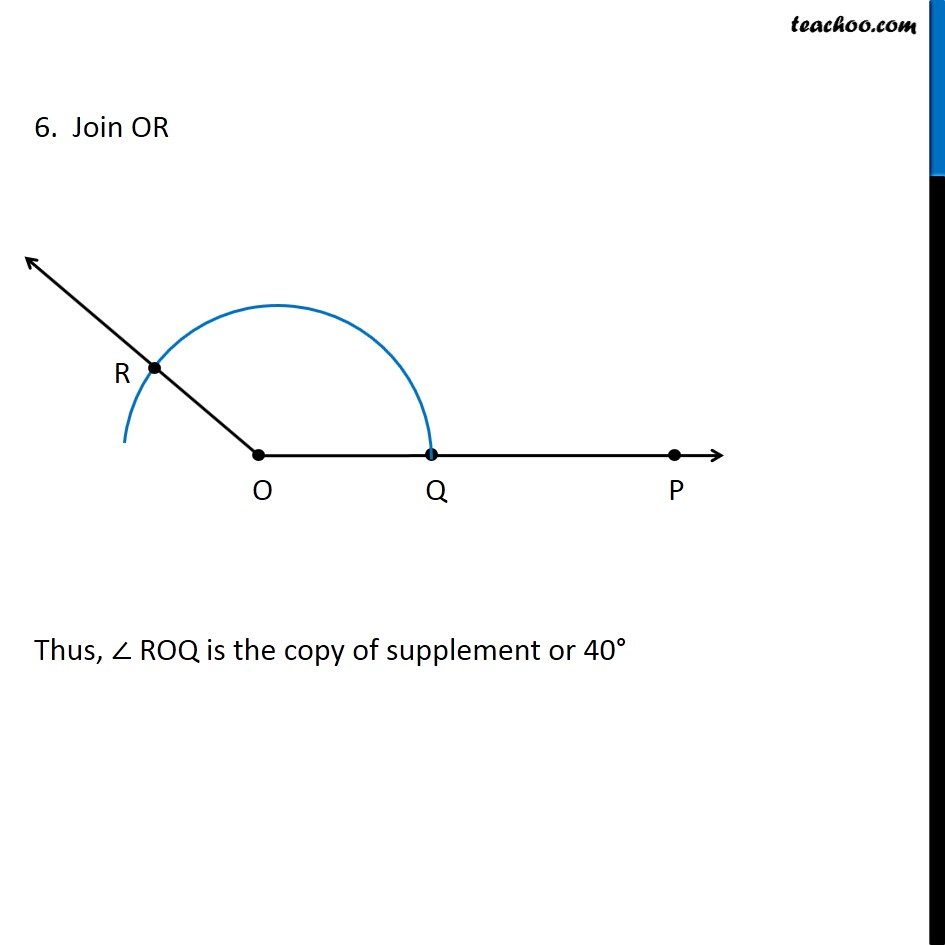Learn in your speed, with individual attention - Teachoo Maths 1-on-1 Class

### Transcript

Question 9 Draw an angle of 40 °. Copy its supplementary angle. Let’s first draw a rough figure So, lets first draw 40° We follow these steps Draw a line with point O & A on it 2. Place center of protractor on point O, and coincide line OA and Protractor line Mark point B on 40° Join OB ∴ ∠ AOB = 40° Now, we need to make a copy of its supplement. Let’s follow these steps Draw a ray OP 2. In our ∠ AOB, From point O, draw an arc of any radius on the supplement of 40°, intersecting the sides at D & E 3. Using compass opened the same length, draw an arc with point O as the center, cutting OP at Q 4. Now, back to our angle ∠ BOA. Open compass to length DE 5. Keeping the same radius, Put pointed end of compass on point Q, and draw an arc. The point where it cuts previous arc is point R. 6. Join OR Thus, ∠ ROQ is the copy of ∠ BOA = 70° 6. Join OR Thus, ∠ ROQ is the copy of ∠ BOA = 70°